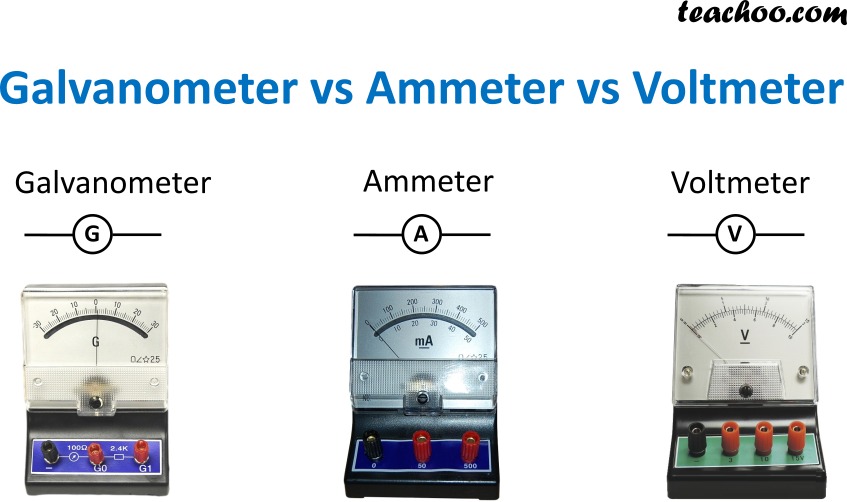Galvanometer Ammeter Voltmeter It is an instrument used to detect the flow of current in the circuit. It is an instrument used to show the amount of current in the circuit. It is an instrument used to show the amount of potential difference across two points in the circuit. It tells the direction of current in the circuit. It doesn’t tell about the direction of current in the circuit. It tells nothing about the current in the circuit. It is connected in series in a circuit. It is connected in series in a circuit. It is connected in parallel in a circuit. It has moderate resistance (10-100 ohms) An ideal ammeter should have zero resistance, hence resistance of an ammeter is very small. An ideal voltmeter should have infinite resistance, hence resistance of a voltmeter is very high. Magnetic Field is required for Galvanometer to work Ammeter can work with or without magnetic field Voltmeter can work with or without magnetic field It measures only direct current It measures both direct and alternating current It tells nothing about current in the circuit

1. Class 10
2. Chapter 13 Class 10 - Magnetic Effects of Electric Current
3. Extra Questions

Extra Questions

Class 10
Chapter 13 Class 10 - Magnetic Effects of Electric Current